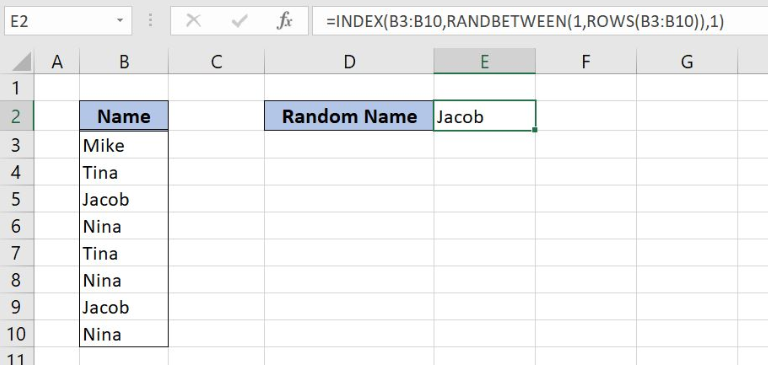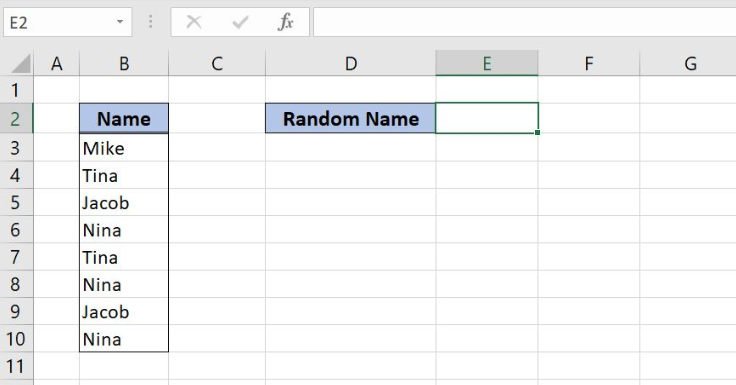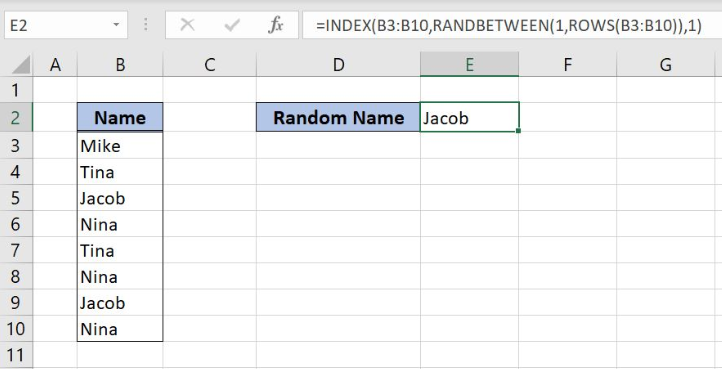Get instant live expert help with Excel or Google Sheets“My Excelchat expert helped me in less than 20 minutes, saving me what would have been 5 hours of work!”

#### Post your problem and you'll get expert help in seconds

Your message must be at least 40 characters
Our professional experts are available now. Your privacy is guaranteed.

# Random Value From List or TableRead time: 20 minutes

Excel allows us to get a random value from a list or table, by using the INDEX, RANDBETWEEN and ROWS functions. This step by step tutorial will assist all levels of Excel users in understanding how to get a random value from the list using these 3 functions.Figure 1. The final result of the functions

## Syntax of the INDEX formula

`=INDEX(array, row_num, [column_num])`

The parameters of the INDEX function are:

• array – a list of values where we want to find a value from a certain position
• row_num – a row from which we want to get a value
• [column_num] – a column from which we want to get a value. It’s a non-mandatory parameter and if it’s omitted it’s by default 1.

## Syntax of the RANDBETWEEN formula

`=RANDBETWEEN(bottom, top)`

The parameters of the RANDBETWEEN function are:

• bottom – a value from which we want to get a random value
• top – a value to which we want to get a random value

## Syntax of the ROWS formula

`=ROWS(array)`

The parameter of the ROW function is:

• array – an array for which we want to get the total number of rows.

## Setting up Our Data for the Functions

In column B (“Names”), we have the list of names for which we want to find the random name. The result will be stored in the cell E3Figure 2. Data that we will use in the example

## Get a Random Name from the List of Names

In the cell E3, we want to get the random name from the list of names in the range B3:B10.

The formula looks like:

`=INDEX(B3:B10, RANDBETWEEN(1, ROWS(B3:B10)), 1)`

The array is B3:B10. The row_num is RANDBETWEEN(1, ROWS(B3:B10)), while the column_num is 1.

To apply the formula, we need to follow these steps:

• Select cell E3 and click on it
• Insert the formula: `=INDEX(B3:B10, RANDBETWEEN(1, ROWS(B3:B10)), 1)`
• Press enter.Figure 3. Using the INDEX function to get a random name from the list

The INDEX function searches the B3:B10 range. The row_num is the result of the RANDBETWEEN function, which returns a random number between 1 and 8. In this example, this function returned 3, so the INDEX returns the value from the third row. Therefore, the result of the formula in the cell E3 is “Jacob”.

Most of the time, the problem you will need to solve will be more complex than a simple application of a formula or function. If you want to save hours of research and frustration, try our live Excelchat service! Our Excel Experts are available 24/7 to answer any Excel question you may have. We guarantee a connection within 30 seconds and a customized solution within 20 minutes.

### Did this post not answer your question? Get a solution from connecting with the expert.Another blog reader asked this question today on Excelchat:
Related blogs## Subscribe to Excelchat.coAnother blog reader asked this question today on Excelchat: